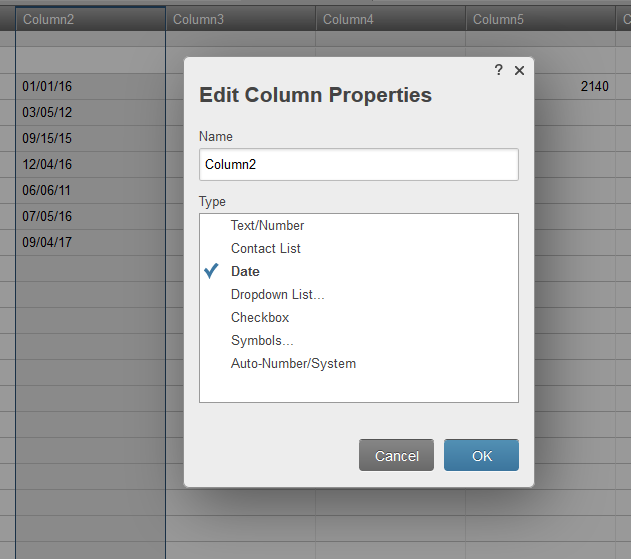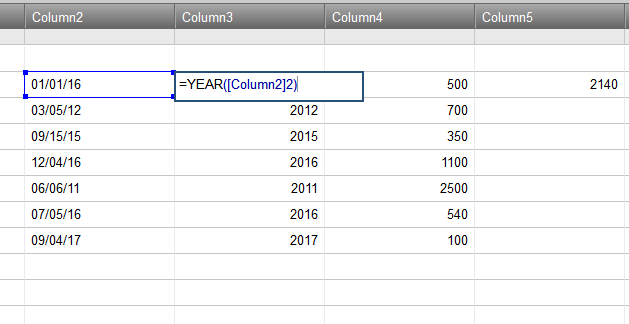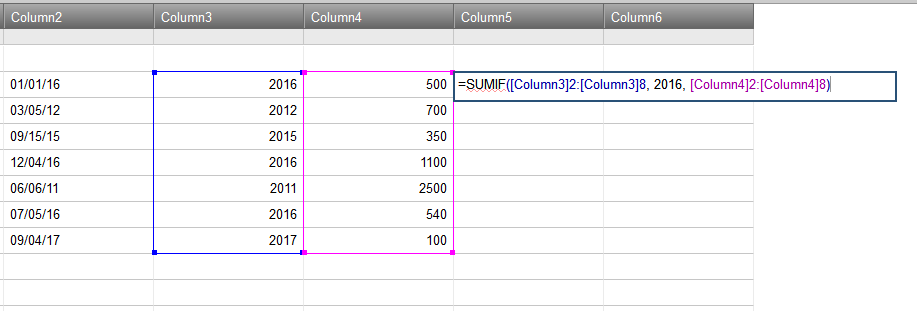#### Welcome to the Smartsheet Forum Archives

The posts in this forum are no longer monitored for accuracy and their content may no longer be current. If there's a discussion here that interests you and you'd like to find (or create) a more current version, please Visit the Current Forums.

# SUMIF YEAR

Hi,

I would like to do:

SUMIF(YEAR(A1:A50), 2016, B1:B50)

The above doesn't work. How else can I construct this formula? thanks,

Column A is a date column

Column B is a currency/number column

Tags:

• What you should do is have a separate column for the Year, unfortunately . I am not aware of anything that can give you YEAR(range) capabilities.

• Thank you for the suggestion Matt.

• You could also use the @cell function so you don't have to create another column for the year. I tested an example SUMIF formula which seems to work in my sheet:

=SUMIF([Start Date]1:[Start Date]7, YEAR(@cell) = 2016, Cost1:Cost7)

You'll want to change the cell references to the column names of your own sheet. More on the @cell in our Help Center: http://help.smartsheet.com/articles/775363-using-formulas#at_cell

• Wow. That is much nicer.

Thanks!

• Thanks Shaine. I'm getting INVALID DATA TYPE error when I add this.

Please note that the date column itself is calculated based on a formula. The way it works is that:

• * I have a column that captures the date of deployment of an item (DATE OF DEPLOYMENT)

* another column that captures the life of the item in years (LIFE IN YEARS),

* then an END OF LIFE column that adds the number of years to the date of deployment and gives me the year the product will expire. The formula I have in END OF LIFE is:

=IF(ISNUMBER([Life in Years]41), DATE(YEAR([Date of Deployment]41) + [Life in Years]41, MONTH([Date of Deployment]41), DAY([Date of Deployment]41)), " ")

There is a Price column for each item. So the SUMIF formula is to calculate the total price of products that'll expire in the END OF LIFE year. For 2016 I have:

=SUMIF([End of Life]36:[End of Life]70, YEAR(@cell) = 2016, [Price]36:[Price]70)

This is where I'm getting a error.

Hope this makes sense.

• Another layer of complexity is that our financial year is from October to September. So for 2016 - the condition for SUMIF should include YEAR(@cell)=2016 AND Month(@cell) is 10/2016 to 9/2017. Is this doable?

• To add more than one condition to a SUM you would want to use a SUMIFS instead of SUMIF. Using Shaine's example you would go from this:

=SUMIF([Start Date]1:[Start Date]7, YEAR(@cell) = 2016, Cost1:Cost7)

to:

=SUMIFS(Cost1:Cost7, [Start Date]1:[Start Date]7, YEAR(@cell) = 2016 , [Start Date]1:[Start Date]7, @CELL >= DATE(2016,10,01), [Start Date]1:[Start Date]7, @CELL <= DATE(2017,09,30))

My question would be then, do you need the YEAR(@cell) = 2016 if you give a hard date range? It sounds like you wouldn't. Additionally it sounds like you want to replace Start Date with End of Life. And by doing that I think you would have to apply your IF(ISNUMBER.... formula into the SUMIFS formula instead of referencing the End of Life column.

• @Matt I don't think you'll need the YEAR(@cell) = 2016 if you're providing a hard DATE(xxxx, xx, xx) comparison in the formula.

@Meenakshi you'll get the #INVALID DATA TYPE error typically if the formula is placed in an incompatible column, or it's referencing an incompatible column. Check the column type by right clicking the column header at the top.

SUMIF columns typically work best when placed in text/number columns, and the sum range typically should be a text/number column.

If your date column isn't actually a date type, the formula will return the error due to the YEAR() function.

• I was able to make it work by setting a separate column to generate the year from the date column. Interestingly enough, SUMIF didn't work when the YEAR() function was embedded in it. See the attached screen shots. Hope it helps.• Thank you everyone for helping me understand the logic and providing a solution. @CELL&gt;=DATE(xxxx,xx,xx) didn't work though. Just >=DATE(...) worked fine. Super, thank you again.

This discussion has been closed.